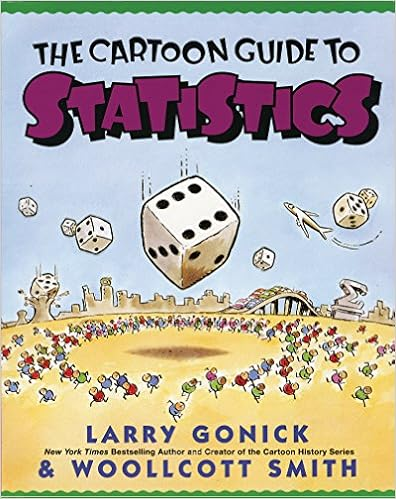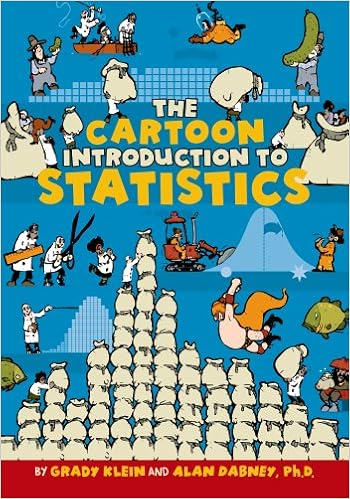# Math for Intelligence

A brief guide about how to minimize a function with millions of variables

There are three kinds of lies: lies, damned lies, and statistics. - Mark Twain

# Math for Intelligence - Getting Started

 Math is the hidden secret to understanding the world | Roger Antonsen Unlock the mysteries and inner workings of the world through one of the most imaginative art forms ever -- mathematics -- with Roger Antonsen, as he explains how a slight change in perspective can reveal patterns, numbers and formulas as the gateways to empathy and understanding. I am a logician, mathematician, computer scientist, author, public speaker, science communicator, and artist. You can find me at the University of Oslo, where I teach Logical Methods as an Associate Professor at the Department of Informatics in the research group Analytical Solutions and Reasoning (ASR), otherwise at UC Berkeley, California and ICERM at Brown University, where I am a Visiting Scholar. I am also engaged in various forms of science communication and outreach, which you may read about below. My academic interests are logical calculi, proof theory, mathematical logic, complexity theory, automata, combinatorics, philosophy of mathematics, visualizations, and mathematical art, but I am interested in most topics related to mathematics, computer science, art, and philosophy.
 Mathematics is the sense you never knew you had | Eddie Woo | TEDxSydney In this illuminating talk, high school mathematics teacher and YouTube star Eddie Woo shares his passion for mathematics, declaring that "mathematics is a sense, just like sight and touch" and one we can all embrace. Using surprising examples of geometry, he encourages everyone to seek out the patterns around us, for "a whole new way to see the world". A public high school teacher for more than 10 years, Eddie Woo gained international attention when he posted videos of his classroom lessons online, to assist an ill student. His YouTube channel, WooTube, has more than 200,000 subscribers and over 13 million views. Eddie believe that mathematics can be embraced and even enjoyed by absolutely everybody. He was named Australia's Local Hero and was a Top 10 Finalist in the Global Teacher Prize for his love of teaching mathematics. This talk was given at a TEDx event using the TED conference format but independently organized by a local community. Learn more at https://www.ted.com/tedx
 How you can be good at math, and other surprising facts about learning | Jo Boaler | TEDxStanford You have probably heard people say they are just bad at math, or perhaps you yourself feel like you are not “a math person.” Not so, says Stanford mathematics education professor Jo Boaler, who shares the brain research showing that with the right teaching and messages, we can all be good at math. Not only that, our brains operate differently when we believe in ourselves. Boaler gives hope to the the mathematically fearful or challenged, shows a pathway to success, and brings into question the very basics of how our teachers approach what should be a rewarding experience for all children and adults. Jo Boaler is a professor of mathematics education at Stanford and the co-founder of YouCubed, which provides resources and ideas to inspire and excite students about mathematics. She is also the author of the first massive open online course on mathematics teaching and learning. Her book Experiencing School Mathematics won the Outstanding Book of the Year award for education in Britain. A recipient of a National Science Foundation "early career award"' she was recently named by BBC as one of the eight educators changing the face of education. This talk was given at a TEDx event using the TED conference format but independently organized by a local community. Learn more at http://ted.com/tedx
 Love and Math an interview with Edward Frenkel UC Professor of mathematics Edward Frenkel describes the relationship of Love and Mathematics, calls for a more modern way of teaching math in schools, and talks of the principles and people that have advanced our understanding of Math as a window onto reality. Edward Frenkel is a professor of mathematics at the University of California, Berkeley, which he joined in 1997 after being on the faculty at Harvard University. He is a member of the American Academy of Arts and Sciences, a Fellow of the American Mathematical Society, and the winner of the Hermann Weyl Prize in mathematical physics. Frenkel has authored three books and over eighty scholarly articles in academic journals, and he has lectured on his work around the world. His YouTube videos have garnered over 4 million views combined. Frenkel’s latest book Love and Math was a New York Times bestseller and has been named one of the Best Books of the year by both Amazon and iBooks. It is being translated into 16 languages. Frenkel has also co-produced, co-directed and played the lead in the film Rites of Love and Math (2010).

## Mathematics Ontology

 The Map of Mathematics The entire field of mathematics summarised in a single map! This shows how pure mathematics and applied mathematics relate to each other and all of the sub-topics they are made from.
 Mind Map of Maths LarryLemonMaths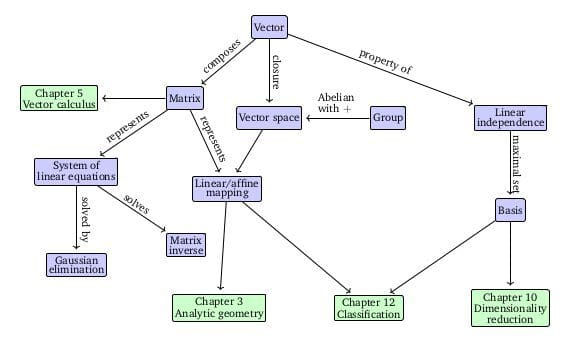The Roadmap of Mathematics for Deep Learning | Tivadar Danka ...Understanding the inner workings of neural networks from the ground-up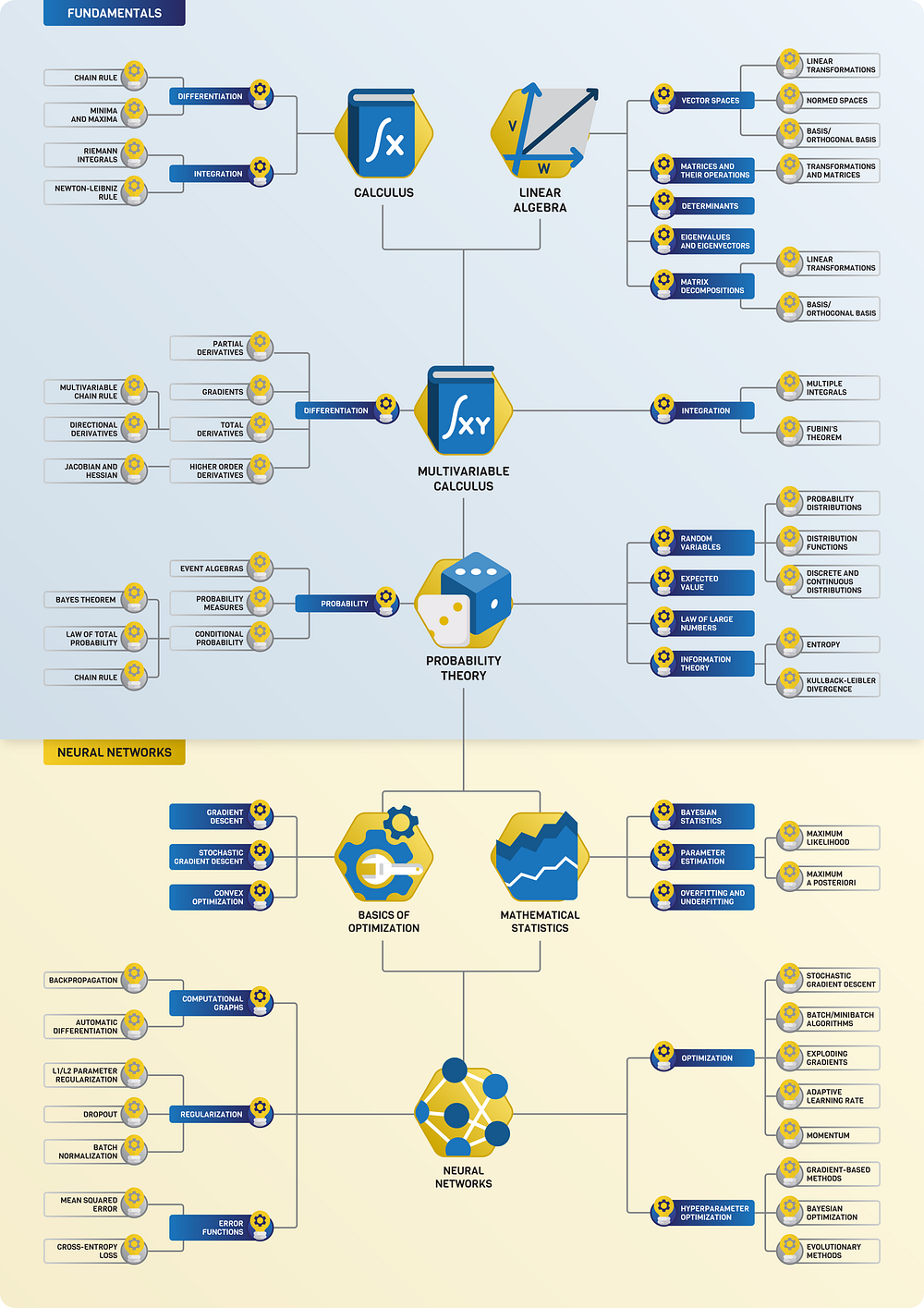## Scalar, Vector, Matrix & TensorHow Data travels in Deep Neural Networks | Scalar vs Vector vs Matrix vs Tensor This video titled "How Data travels in Deep Neural Networks | Scalar vs Vector vs Matrix vs Tensor" explains the role of Tensors in TensorFlow, What exactly are tensors as well as Characteristics of tensors. How Data travels in Deep Neural Networks? A comparison of Scalar vs Vector vs Matrix vs Tensor. SUPPORT ME on Patreon: http://www.patreon.com/theaiuniversity
 Why Linear Algebra ? Scalars, Vectors, Tensors NPTEL-NOC IITM Lecture - 03
 Transformation properties of scalars, vectors and tensors This video is on the basics of scalars, vectors and tensors and their transformation properties.
 Scalar, Vector, Matrix, Tensor, Matrix Transpose Deep Shallownet Topics to cover based on: Deep Learning An MIT Press book Ian Goodfellow and Yoshua Bengio and Aaron Courville http://www.deeplearningbook.org/

### Scalars

a single number. For example weight, which is denoted by just one number.

### Vector

Vectors are an array of numbers. The numbers are arranged in order and we can identify each individual number by its index in that ordering. We can think of vectors as identifying points in space, with each element giving the coordinate along a different axis. In simple terms, a vector is an arrow representing a quantity that has both magnitude and direction wherein the length of the arrow represents the magnitude and the orientation tells you the direction. For example wind, which has a direction and magnitude.

 What is a vector? - David Huynh Physicists, air traffic controllers, and video game creators all have at least one thing in common: vectors. But what exactly are they, and why do they matter? David Huynh explains how vectors are a prime example of the elegance, beauty, and fundamental usefulness of mathematics. Lesson by David Huynh, animation by Anton Trofimov.

### Matrices

A matrix is a 2D-array of numbers, so each element is identified by two indices instead of just one. If a real valued matrix A has a height of m and a width of n, then we say that A in Rm x n. We identify the elements of the matrix as A_(m,n) where m represents the row and n represents the column.

 The Applications of Matrices | What I wish my teachers told me way earlier Zach Star This video goes over just a few applications of matrices that may give you some insight into how they can be used in the real world. Linear algebra was never explained well to me in school and I had very little motivation to learn matrices at the time so hopefully this helps you if you're in a similar situation. Also note there are so many applications there's just no way to fit them into one video but here you'll find some of my favorite instances of when they come up. Support the Channel: http://www.patreon.com/zachstar Google PageRank Algorithm: http://youtu.be/qxEkY8OScYY Mathematics Used to Solve Crime: http://youtu.be/-cXBgHgX5UE
 Dear linear algebra students, This is what matrices (and matrix manipulation) really look like Sign up with brilliant and get 20% off your annual subscription: http://brilliant.org/ZachStar/ Support the Channel: http://www.patreon.com/zachstar 3D Software Used Runiter (for the actual graphs): http://www.runiter.com/ Geogebra (used for vectors, & is free): http://www.geogebra.org/3d?lang=en Animations: Brainup Studios ( http://brainup.in/ )
 Multiplying Matrices This precalculus video tutorial provides a basic introduction into multiplying matrices. It explains how to tell if you can multiply two matrices together and how to determine the order of the new matrix. The order of the new matrix is based on the rows of the first matrix and the number of columns in the second matrix. To multiply two matrices together, multiply the rows of the first matrix by the columns of the second matrix and find the sum of the products. This video contains plenty of examples and practice problems on matrix multiplication. My E-Book: https://amzn.to/3B9c08z Video Playlists: https://www.video-tutor.net Homework Help: https://bit.ly/Find-A-Tutor
 How to organize, add and multiply matrices - Bill Shillito When you're working on a problem with lots of numbers, as in economics, cryptography or 3D graphics, it helps to organize those numbers into a grid, or matrix. Bill Shillito shows us how to work with matrices, with tips for adding, subtracting and multiplying (but not dividing!). Lesson by Bill Shillito, animation by The Leading Sheep Studios.

### Tensors

In mathematics, a tensor is an algebraic object that describes a (multilinear) relationship between sets of algebraic objects related to a vector space. Objects that tensors may map between include vectors and scalars, and, recursively, even other tensors. Tensors can take several different forms – for example: scalars and vectors (which are the simplest tensors), dual vectors, multi-linear maps between vector spaces, and even some operations such as the dot product. Tensors are defined independent of any basis, although they are often referred to by their components in a basis related to a particular coordinate system. Wikipedia

 What's a Tensor? Dan Fleisch briefly explains some vector and tensor concepts from A Student's Guide to Vectors and Tensors
 Tensors Explained Intuitively: Covariant, Contravariant, Rank Tensors of rank 1, 2, and 3 visualized with covariant and contravariant components. My Patreon page is at http://www.patreon.com/EugeneK

## 3blue1brown

 Gradient descent, how neural networks learn | Deep learning, chapter 2 Home page: http://www.3blue1brown.com/ Brought to you by you: http://3b1b.co/nn2-thanks And by Amplify Partners. For any early-stage ML startup founders, Amplify Partners would love to hear from you via 3blue1brown@amplifypartners.com To learn more, I highly recommend the book by Michael Nielsen http://neuralnetworksanddeeplearning.com/ The book walks through the code behind the example in these videos, which you can find here: http://github.com/mnielsen/neural-networks-and-deep-learning MNIST database: http://yann.lecun.com/exdb/mnist/ Also check out Chris Olah's blog: http://colah.github.io/ His post on Neural networks and topology is particular beautiful, but honestly all of the stuff there is great. And if you like that, you'll *love* the publications at distill: http://distill.pub/
 What is backpropagation really doing? | Deep learning, chapter 3 What's actually happening to a neural network as it learns? Next video: http://youtu.be/tIeHLnjs5U8 Brought to you by you: http://3b1b.co/nn3-thanks And by CrowdFlower: http://3b1b.co/crowdflower Home page: http://www.3blue1brown.com/ The following video is sort of an appendix to this one. The main goal with the follow-on video is to show the connection between the visual walkthrough here, and the representation of these "nudges" in terms of partial derivatives that you will find when reading about backpropagation in other resources, like Michael Nielsen's book or Chis Olah's blog.
 Backpropagation calculus | Deep learning, chapter 4 Brought to you by you: http://3b1b.co/nn3-thanks This one is a bit more symbol heavy, and that's actually the point. The goal here is to represent in somewhat more formal terms the intuition for how backpropagation works in part 3 of the series, hopefully providing some connection between that video and other texts/code that you come across later. 3blue1brown is a channel about animating math, in all senses of the word animate. And you know the drill with YouTube, if you want to stay posted on new videos, subscribe, and click the bell to receive notifications (if you're into that): http://3b1b.co/subscribe If you are new to this channel and want to see more, a good place to start is this playlist: http://3b1b.co/recommended

### Determinants

the determinant of a matrix describes how the volume of an object scales under the corresponding linear transformation. If the transformation changes orientations, the sign of the determinant is negative.

 The determinant | Essence of linear algebra, chapter 6 Home page: http://www.3blue1brown.com/ The determinant of a linear transformation measures how much areas/volumes change during the transformation. Full series: http://3b1b.co/eola Future series like this are funded by the community, through Patreon, where supporters get early access as the series is being produced. http://3b1b.co/support 3blue1brown is a channel about animating math, in all senses of the word animate. And you know the drill with YouTube, if you want to stay posted about new videos, subscribe, and click the bell to receive notifications (if you're into that). If you are new to this channel and want to see more, a good place to start is this playlist: http://goo.gl/WmnCQZ Various social media stuffs: Website: http://www.3blue1brown.com

## Explained

 The Mathematics Of Intelligence | Geoff Goodhill | TEDxUQ Professor Goodhill's research aims to discover the computational rules underlying brain development and function. He originally trained in the UK in maths, physics and artificial intelligence, and then spent 10 years researching in the USA, including 8 as a professor of neuroscience at Georgetown University. He moved to the University of Queensland in 2005, where he holds a joint appointment between the Queensland Brain Institute and School of Mathematics and Physics. His lab uses experimental, mathematical and computational techniques to understand the brain as a computational device. Professor Goodhill did a Joint Honours BSc in Mathematics and Physics at Bristol University (UK), followed by an MSc in Artificial Intelligence at Edinburgh University and a PhD in Cognitive Science at Sussex University. Following a postdoc at Edinburgh University he moved to the USA in 1994, where he did further postdoctoral study in Computational Neuroscience at Baylor College of Medicine and the Salk Institute. Professor Goodhill formed his own lab at Georgetown University in 1996, where he was awarded tenure in the Department of Neuroscience in 2001. In 2005 he moved to a joint appointment between the Queensland Brain Institute and the School of Mathematical and Physical Sciences at the University of Queensland. This talk was given at a TEDx event using the TED conference format but independently organized by a local community. Learn more at http://ted.com/tedx
 Connections between physics and deep learning MITCBMM Max Tegmark - MIT

## Gilbert Strang (MIT) - Linear Algebra

 Course Introduction | MIT 18.06SC Linear Algebra Instructor: Gilbert Strang View the complete course: http://ocw.mit.edu/18-06SCF11 Professor Gil Strang describes the key concepts of undergraduate course Linear Algebra, who should take it, and how it is taught. He provides examples of applications of linear algebra and how it is useful in physics, economics and social sciences, natural sciences, and engineering. License: Creative Commons BY-NC-SA More information at http://ocw.mit.edu/terms More courses at http://ocw.mit.edu
 A conversation with Gilbert Strang Gilbert Strang was an undergraduate at MIT and a Rhodes Scholar at Balliol College, Oxford. His Ph.D. was from UCLA and since then he has taught at MIT. He has been a Sloan Fellow and a Fairchild Scholar and is a Fellow of the American Academy of Arts and Sciences. He is a Professor of Mathematics at MIT, an Honorary Fellow of Balliol College, and a member of the National Academy of Sciences. Professor Strang has published eleven books.

## Fourier Transform (FT), Fourier Series, and Fourier Analysis

Joseph Fourier showed that representing a function as a sum of trigonometric functions greatly simplifies the study of heat transfer. Joseph was a French mathematician and physicist born in Auxerre and best known for initiating the investigation of Fourier series, which eventually developed into Fourier analysis and harmonic analysis, and their applications to problems of heat transfer and vibrations. The Fourier transform and Fourier's law of conduction are also named in his honor. Fourier is also generally credited with the discovery of the greenhouse effect.

• Fourier Transform (FT) decomposes a function of time (a signal) into its constituent frequencies. This is similar to the way a musical chord can be expressed in terms of the volumes and frequencies of its constituent notes. Fourier Transform | Wikipedia
• Fourier Series is a periodic function composed of harmonically related sinusoids, combined by a weighted summation. The discrete-time Fourier transform is an example of Fourier series. For functions on unbounded intervals, the analysis and synthesis analogies are Fourier Transform and inverse transform. Fourier Series | Wikipedia
• Fourier Analysis the study of the way general functions may be represented or approximated by sums of simpler trigonometric functions. Fourier Analysis | WikipediaWhat is a Fourier Series? (Explained by drawing circles) - Smarter Every Day 205 Doga's a super smart dude who writes a Turkish blog "Bi Lim Ne Güzel Lan" that roughly translates roughly to "Science is Awesome Dude". We had a lot of fun working on this together. He would really appreciate it if you checked out his blog. The fun thing is that most of his articles transcend language. Doga’s Blog (written in Turkish): http://bilimneguzellan.net/ Doga’s original Fourier Series blog article that blew my mind: http://bilimneguzellan.net/fuyye-serisi/ Click here to tweet him "thanks" for http://twitter.com/bilimneguzellan Get a free crate for a kid you love (Awesome Chrsitmas gifts) at: http://www.kiwico.com/smarter Click here if you're interested in subscribing: http://bit.ly/Subscribe2SED Brady’s Video “Optical Tweezers and the 2018 Nobel Prize in Physics - Sixty Symbols” http://www.youtube.com/watch?v=XjXLJMUrNBo
 Fourier Transform, Fourier Series, and frequency spectrum Physics Videos by Eugene Khutoryansky Fourier Series and Fourier Transform with easy to understand 3D animations.
 But what is the Fourier Transform? A visual introduction An animated introduction to the Fourier Transform. Home page: http://www.3blue1brown.com/ Brought to you by you: http://3b1b.co/fourier-thanks Follow-on video about the uncertainty principle: http://youtu.be/MBnnXbOM5S4 Animations largely made using manim, a scrappy open-source Python library. http://github.com/3b1b/manim If you want to check it out, I feel compelled to warn you that it's not the most well-documented tool, and has many other quirks you might expect in a library someone wrote with only their own use in mind.
 Fourier Analysis For The Rest Of Us GoldPlatedGoof http://twitter.com/goldplatedgoof

# Mathematical Reasoning

Large Language Model (LLM)s have limited performance when solving arithmetic reasoning tasks and often provide incorrect answers. Unlike Natural Language Understanding (NLU), math problems typically have a single correct answer, making the task of generating accurate solutions more challenging for LLMs. However, there are techniques being developed to improve the performance of LLMs on arithmetic problems.

• For example, `MathPrompter` is a technique that improves the performance of LLMs on arithmetic problems along with increased reliance in the predictions. MathPrompter uses the Zero-shot Chain of Thought (CoT) prompting technique to generate multiple Algebraic expressions or Python functions to solve the same math problem in different ways and thereby raise the confidence level in the output results. MathPrompter thus leverages solution-verification approaches such as those used by humans — compliance with known results, multi-verification, cross-checking and compute verification — to increase confidence in its generated answers. The MathPrompter pipeline comprises four steps. Given a question:
1. An algebraic template is generated, replacing the numerical entries with variables;
2. The LLM is fed multiple math prompts that can solve the generated algebraic expression analytically in different ways;
3. The analytical solutions are evaluated by allotting multiple random values to the algebraic expression; and
4. A statistical significance test is applied to the solutions of the analytical functions to find a “consensus” and derive the final solution³.
• Another technique is Algorithmic Prompting, a new method of prompt engineering for Large Language Model (LLM)s The method gives a model a detailed algorithm for solving a math problem. With algorithmic prompting, the math performance of language models increases by up to ten times. Algorithmic Prompting` involves providing a detailed description of the algorithm execution on running examples, and using explicit explanation and natural language instruction to remove ambiguity. For example, using addition as an example, the team behind this technique shows that large language models can apply instructions with as few as five digits to as many as 19 digits. This is an example of out-of-distribution generalization and a direct effect of algorithmic prompting.

# Math Mistakes | Matt Parker

 The Greatest Maths Mistakes | Matt Parker | Talks at Google When math goes wrong, things can get expensive. Or absolutely hilarious. For this talk we invited YouTube personality (Numberphile, standupmaths), math communicator, comedian, and one third of the Festival of the Spoken Nerd, Matt Parker, to share his favorite math mistakes from his new UK #1 bestseller, "Humble Pi - A Comedy of Maths Errors". Matt exposes errors on the Two Pound Coin, very specific rules for trains operating in Switzerland, and how simple unit conversion slip ups can cost billions of dollars. He also discusses the infamous 256th level of Pac-Man and answers audience questions about more hilarious mathematical failures. Get the book here: http://goo.gl/G4kqw6
 What Happens When Maths Goes Wrong? - with Matt Parker Most of the time, the maths in our everyday lives works quietly behind the scenes, until someone forgets to carry a '1' and a bridge collapses or a plane drops out of the sky. Subscribe for regular science videos: http://bit.ly/RiSubscRibe Matt's book "Humble Pi" available now: https://geni.us/9nPhpn3 Matt Parker is a stand-up comedian and mathematician. He appears regularly on TV and online: as well as being a presenter on the Discovery Channel. His YouTube videos have been viewed over 37 million times. Previously a high-school mathematics teacher, Matt visits schools to talk to students about maths as part of Think Maths and he is involved in the Maths Inspiration shows. In his remaining free time, Matt wrote the books Things To Make and Do in the Fourth Dimension and Humble Pi: A Comedy of Maths Errors. He is also the Public Engagement in Mathematics Fellow at Queen Mary University of London. This talk was filmed in the Ri on 1 March 2019.

# Statistics for Intelligence

There are lies, damned lies and statistics. - Mark Twain

## Data Representation

### Stem and Leaf Plot

a special table where each data value is split into a "stem" (the first digit or digits) and a "leaf" (usually the last digit). | Math is Fun A stem and leaf plot is a great way to organize data by the frequency. It is a great visual that also includes the data. So if needed, you can just take a look to get an idea of the spread of the data or you can use the values to calculate the mean, median or mode. SoftSchools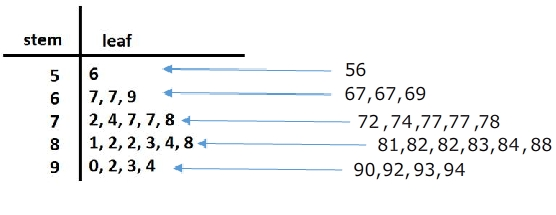### Histograms

Histograms are one of the most basic statistical tools that we have. They are also one of the most powerful and most frequently used.

### Mean, Median, and Mode

• Mean : The sum of all the data divided by the number of data sets. Example: 8 + 7 + 3 + 9 + 11 + 4 = 42 ÷ 6 = Mean of 7.0
• Median : The mid data point in a data series organised in sequence. Example : 2 5 7 8 11 14 18 21 22 25 29 (five data values either side)
• Mode : The most frequently occurring data value in a series. Example : 2 2 4 4 4 7 9 9 9 9 12 12 13 ( ‘9’ occurs four times, so is the ‘mode’)

### Interquartile Range (IQR)

The interquartile range is a measure of where the “middle fifty” is in a data set. Where a range is a measure of where the beginning and end are in a set, an interquartile range is a measure of where the bulk of the values lie. That’s why it’s preferred over many other measures of spread (i.e. the average or median) when reporting things like school performance or SAT scores. The interquartile range formula is the first quartile subtracted from the third quartile. Interquartile Range (IQR): What it is and How to Find it | Statistics How To

### Box & Whisker Plots (Boxplot)

presents information from a five-number summary especially useful for indicating whether a distribution is skewed and whether there are potential unusual observations (outliers) in the data set. Box and whisker plots are also very useful when large numbers of observations are involved and when two or more data sets are being compared. Constructing box and whisker plots | Statistics Canada### Standard Deviation

Greek letter sigma σ for the population standard deviation or the Latin letter s for the sample standard deviation) is a measure that is used to quantify the amount of variation or dispersion of a set of data values. A low standard deviation indicates that the data points tend to be close to the mean (also called the expected value) of the set, while a high standard deviation indicates that the data points are spread out over a wider range of values. Standard Deviation | Wikipedia

## Probability

Probability is the likelihood or chance of an event occurring. Probability = the number of ways of achieving success. the total number of possible outcomes.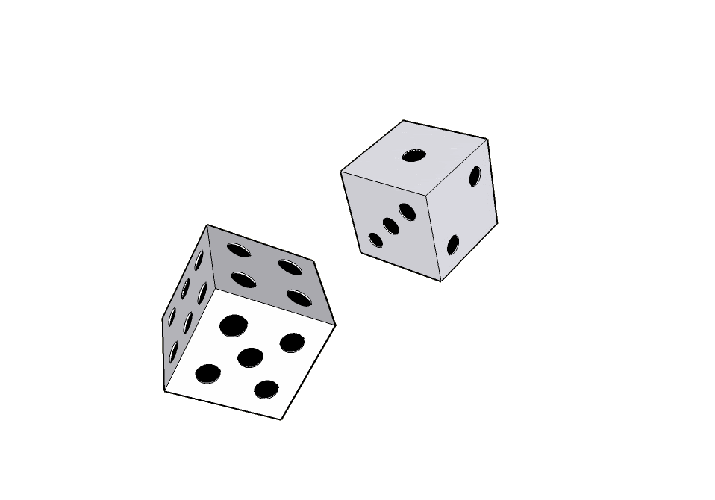### Conditional Probability

the probability of an event ( A ), given that another ( B ) has already occurred.

### Probability Independence

In probability theory, two events are independent, statistically independent, or stochastically independent if the occurrence of one does not affect the probability of occurrence of the other (equivalently, does not affect the odds). Similarly, two random variables are independent if the realization of one does not affect the probability distribution of the other. The concept of independence extends to dealing with collections of more than two events or random variables, in which case the events are pairwise independent if each pair are independent of each other, and the events are mutually independent if each event is independent of each other combination of events. Independence (probability theory) | Wikipedia

## P-Value

In statistics, every conjecture concerning the unknown probability distribution of a collection of random variables representing the observed data X in some study is called a statistical hypothesis. If we state one hypothesis only and the aim of the statistical test is to see whether this hypothesis is tenable, but not, at the same time, to investigate other hypotheses, then such a test is called a significance test. Note that the hypothesis might specify the probability distribution of X precisely, or it might only specify that it belongs to some class of distributions. Often, we reduce the data to a single numerical statistic T whose marginal probability distribution is closely connected to a main question of interest in the study. A statistical hypothesis that refers only to the numerical values of unknown parameters of the distribution of some statistic is called a parametric hypothesis. A hypothesis which specifies the distribution of the statistic uniquely is called simple, otherwise it is called composite. Methods of verifying statistical hypotheses are called statistical tests. Tests of parametric hypotheses are called parametric tests. We can likewise also have non-parametric hypotheses and non-parametric tests. The p-value is used in the context of null hypothesis testing in order to quantify the idea of statistical significance of evidence, the evidence being the observed value of the chosen statistic T. Null hypothesis testing is a reductio ad absurdum argument adapted to statistics. In essence, a claim is assumed valid if its counterclaim is highly implausible. p-value | Wikipedia

 StatQuest: P Values, clearly explained StatQuest with Josh Starmer People often confuse p-values with probabilities. Here I show you how to calculate both and demonstrate their differences. The simple explanation means the concepts are easy to remember. For a complete index of all the StatQuest videos, check out: https://statquest.org/video-index/
 How to calculate p-values StatQuest with Josh Starmer In this StatQuest we learn how to calculate p-values using both discrete data (like coin tosses) and continuous data (like height measurements). At the end, we explain the differences between 1 and 2-sided p-values and why you should avoid 1-sided p-values if possible. For a complete index of all the StatQuest videos, check out: https://statquest.org/video-index/

## Confidence Interval (CI)

In statistics, a confidence interval (CI) is a type of estimate computed from the statistics of the observed data. This proposes a range of plausible values for an unknown parameter (for example, the mean). The interval has an associated confidence level that the true parameter is in the proposed range. Given observations and a confidence level gamma, a valid confidence interval has a probability gamma of containing the true underlying parameter. The level of confidence can be chosen by the investigator. In general terms, a confidence interval for an unknown parameter is based on sampling the distribution of a corresponding estimator. More strictly speaking, the confidence level represents the frequency (i.e. the proportion) of possible confidence intervals that contain the true value of the unknown population parameter. In other words, if confidence intervals are constructed using a given confidence level from an infinite number of independent sample statistics, the proportion of those intervals that contain the true value of the parameter will be equal to the confidence level. For example, if the confidence level (CL) is 90% then in a hypothetical indefinite data collection, in 90% of the samples the interval estimate will contain the population parameter. The confidence level is designated before examining the data. Most commonly, a 95% confidence level is used. However, confidence levels of 90% and 99% are also often used in analysis. Factors affecting the width of the confidence interval include the size of the sample, the confidence level, and the variability in the sample. A larger sample will tend to produce a better estimate of the population parameter, when all other factors are equal. A higher confidence level will tend to produce a broader confidence interval.

 Understanding Confidence Intervals: Statistics Help Dr. Nic's Maths and Stats This short video gives an explanation of the concept of confidence intervals, with helpful diagrams and examples. A good follow-up to check understanding is the video: Confidence Intervals - a quiz to develop understanding. https://youtu.be/gvVD-xlY2Hc See http://creativemaths.net/videos/ for all of Dr. Nic's videos organized by topic.
 StatQuest: Confidence Intervals StatQuest with Josh Starmer A StatQuest http://statquest.org/ for Confidence Intervals. For a complete index of all the StatQuest videos, check out: http://statquest.org/video-index/

## Regression

a statistical technique for estimating the relationships among variables. Regression | Wikipedia

## Analog Computers

Substitute brass for brains - Lord Kelvin; William Thomson, 1st Baron

 The Mechanical Integrator - a machine that does calculus This video explains the function of the mechanical integrator, a mechanism crucial to the development of mechanical analog computers throughout the twentieth century. This video is part of a project I have been working on in collaboration with Professor Michael Littman of Princeton University. One of the goals for developing this specific machine was to use it as a supplementary tool when teaching calculus and differential equations, so I made this video to demonstrate the machine's function with specific emphasis on its connection to calculus. - Jack Monaco
 The Most Powerful Computers You've Never Heard Of Analog computers were the most powerful computers for thousands of years, relegated to obscurity by the digital revolution. This video is sponsored by Brilliant.
 How did the Enigma Machine work? Jared Owen... Let's use 3D animation to go inside the Enigma Machine!
 I Made A Water Computer And It Actually Works Steve Mould... Computers add numbers together using logic gates built out of transistors. But they don't have to be! They can be built out of greedy cup siphons instead! I used specially designed siphones to works as XOR and AND gates and chained them together so they add 4 digit binary numbers.

## Books